## Saturday, November 19, 2011

### Stock levels as delta rows in DAX / BISM Tabular

SQL Server Denali | PowerPivot

A common way to compress large amounts of snap shot data is to store delta values instead of each snapshot value. This makes sense, if the data does not change every day. But for a good query performance you might not want to aggregate data over long periods.Therefore it makes sense to have a regular absolute snapshot value in the data and to use deltas between those snapshots. The work needed to create the periodic snapshots is usually done in the ETL process.

For my example, I’m using the following data table with absolute snapshot values as the first row of the month and deltas afterwards: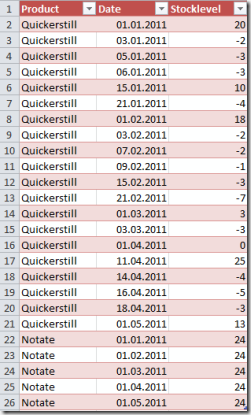For example, for our product Quickerstill we start with a stock level of 20 boxes at the beginning of January 2011. Then, at January 3, 2011 we sold two of them (-2) and again on January 5 another 3 boxes (-3).

The goal is to create a measure that gives us the current stock level at each date (also on the dates between the delta rows).

In order to do so, we need a ‘real’ date dimension, so we have a separate date table that is linked to our facts. This is how this simple model looks like: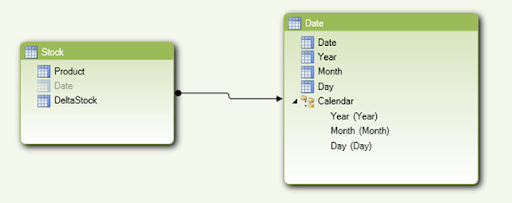The measure DeltaStock is the original stock value from our table above (mixture of snapshots and deltas).

In order to perform the desired computation we can simply use the month-to-date formula as each day’s value computes as the sum from the first day of the month to the current day (including all deltas).

This is the simple formula we’re using to compute the stock at each day:

Stock:=TOTALMTD(SUM([DeltaStock]),'Date'[Date])

It’s amazing how simple this calculation is. Let’s take a look at the result. In order to see the effect, I added the original stock column together with the new computed column.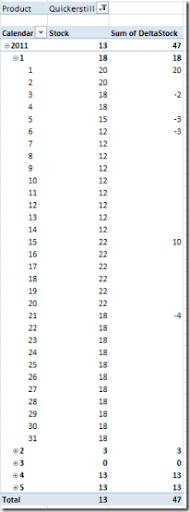As you can see, we now have a stock for each day that computes correctly from the mixture of snapshot and deltas values.

Of course we could also do the calculation without the absolute snapshot values in between. In this case we have to aggregate the values from the very beginning up to the current date. First let’s take a look at the source data without the absolute snapshots: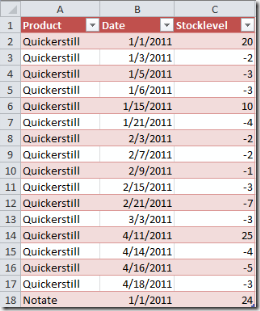The data is pretty much the same as in the first approach. Only the rows for the absolute snapshots are missing (apart from the first initial values).

In this case the calculation would look like this:

Stock:=
SumX(
DATESBETWEEN(
'Date'[Date]
, date(2000,1,1)
, lastdate('Date'[Date])
)
,calculate(
Sum('Stock'[DeltaStock])
,ALLEXCEPT('Date','Date'[Date])
)
)

The calculation is still pretty simple. However I would prefer the option with the snapshot values in between for performance reasons and because these snapshots can be easily created in ETL (if they are not delivered from the source system).

Just two more remarks before I finish this post. The first one is about the DatesBetween range in the formula above. I’m using 2000/1/1 as the start date. However if you take a look at the returned dates only the existing rows from our date table are returned if they are matching this date range. So we could also write 1900/1/1 without risking to end up with a lot of rows.

The other remark is about the future time. Since our calculation takes the last value as the value for all the future, you will find values for all entries of the date dimension. This might not be wanted. In this case you can wrap the calculation from above inside an if statement to check the date:

StockClipped:=
if(
firstdate('Date'[Date])>Now()
, Blank()
,SumX(
DATESBETWEEN(
'Date'[Date]
, date(2000,1,1)
, lastdate('Date'[Date])
)
,calculate(
Sum('Stock'[DeltaStock])
,ALLEXCEPT('Date','Date'[Date])
)
)
)

With this modification (which also works with the formula for the absolute intermediate snapshots from above) values are only shown for periods that are over or have at least started. So the formula would return a value for the full year 2011 once the year has started. If you only want to see values for periods that have ended, you can replace the function ‘firstdate’ with a ‘lastdate’.

## Sunday, October 23, 2011

### Excel Services Scorecard as Windows Desktop Gadget

SharePoint 2010 | SQL Server 2008 | SQL Server 2008R2 | SQL Server 2012 (Denali)

Early this year I stumbled across a very interesting Windows desktop gadget, that is capable of showing an Excel Services element on the Windows desktop. Here, element can be a certain named region, a pivot table or a chart in an Excel Services document. The technology for showing this element is the Excel Services REST API (REST stands for Representational State Transfer).By clicking on the gadget configuration icon, the configuration dialog is displayed: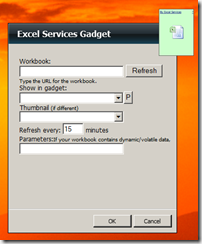Workbook The URL to your Excel Services workbook, for example http://srv1/PowerPivot/Gadget.xlsx Show in gadget Here you can pick from any named region, pivot table or chart that should be displayed in the “expanded” state of the gadget (flyout) Thumbnail Here you can pick from any named region, pivot table or chart that should be displayed in the normal state of the gadget. This is what you see on your desktop first Refresh refresh interval of the gadget

So the simple idea is to create a nice pivot table and to use this as the basis for the gadget. For my example I created a simple pivot table based on the Finance perspective of the Adventure Works OLAP cube. For the pivot table I have the year on the filter, the sales amount and the operating profit KPI as the data and the departments on the rows.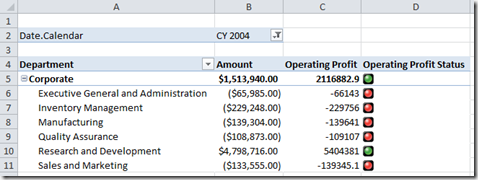If we use this large table in our gadget, the thumbnail view gets pretty much “microscopic”: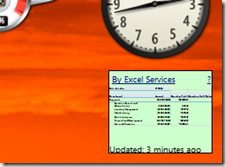So, for the thumbnail (default) view you should choose a much smaller area. The flyout (detail view) is much better, but the KPI indicators and the filters are not shown: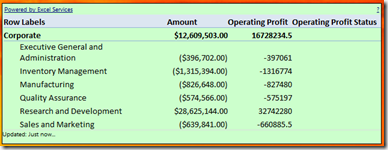So, here are a few tips and tricks you can use to make the gadget look nicer.

1. Use time filters for current year, current month etc.

Of course we want our gadget to always show the latest values. Because of the auto refresh interval, we don’t have to care about this. But usually we will also use a time filter to restrict the data to a specific week, month etc. Since we don’t see the filter, the idea is to have this set automatically to the current time.

This can be easily done in the Excel Pivot Table by using date filters. Unfortunately, these filters don’t work in the filter area, so we have to place the time hierarchy on the rows or columns. Then we can apply a filter (for example current year) as shown here: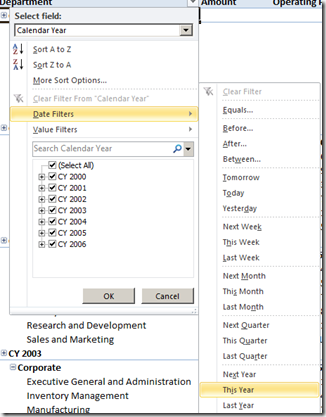For Adventure Works this would not result in any data since the sample date is only available for the years shown in the screenshot. However, in real life scenarios, this would be a good choice for the filter.

2. For thumbnail view, convert the pivot table to formulas

Formulas are much easier to handle and to format, compared to a pivot table. Remembering the very narrow available space for the thumbnail view, having full control of the layout is important. You may even want to hard-code the thumbnail view. Let me explain what I mean. In order to have the connection name at a single place, we start in a blank sheet by putting the connection name in a named cell called “OLAPConnection” (feel free to choose a different name):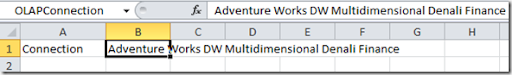As the next step, let’s construct the current date member. From Management Studio, we can see that the MDX name of the time members looks like this (may be different in your cube, this example is taken from the Adventure Works sample database):

• [Date].[Calendar].[Calendar Year].&
• [Date].[Calendar].[Month].&&
• [Date].[Calendar].[Date].&

Here we have January 1, 2001. This is easily constructed using Excel’s time functions. So we simply add these fields to our Excel sheet

 Label/Name Cell Formula Today B3 =Now() Year B4 ="[Date].[Calendar].[Calendar Year].&[" & Year(B3) & "]" Month B5 ="[Date].[Calendar].[Month].&[" & Year(B3) & "]&[" & Month(B3) & "]" Day B6 ="[Date].[Calendar].[Date].&[" & 10000*Year(B3)+100*Month(B3)+Day(B3) &"]"

Of course you could also add fields for the previous month, the week etc., just depending on the needs of your scorecard. I named the cells B4 as MDXYear, B5 as MDXMonth, B6 as MDXDay. This is how the result looks like: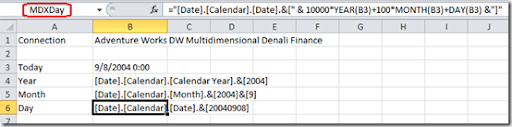I had to fake the current date in order to see some values. Therefore I replaced the formula for today with this one:

=Date(2004, Month(Now()), Day(Now()))

Of course, you won’t want to do this in a real life scenario but since the sample dataset contains no data for 2011 I had to use this “time machine formula”.

Before you start wondering what all this is good for, let’s query some data. For example, let’s assume that we want to see the operating profit (which is on the account ‘Operating Profit’) for the current year. So this would by our Excel formula:

=CUBEVALUE(OLAPConnection,"[Measures].[Amount]","[Account].[Accounts].[Operating Profit]",MDXYear)

In order to show this value in our gadget, I placed it on a new sheet and adjusted the column width and height a little bit.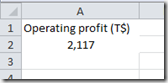In order to get a nice flyout, I also created a simple pivot chart in the Excel sheet, showing the operational profit during the year. After saving the Excel file, our gadget now looks like this: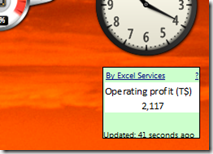And here is the flyout: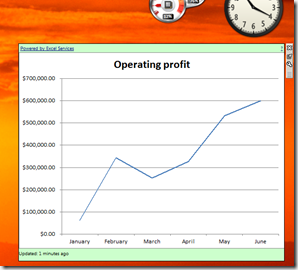3. (Conditional) background colors and fonts are preserved in the REST API

In order to include a kind of traffic light approach, we already found out that Excel indicators are not yet supported in the REST API. However, conditional formatting is supported, so you can easily create a scorecard like the follow: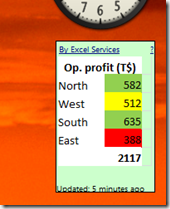Also KPI indicators can be created using special characters, for example from the WingDings font as shown in the following example: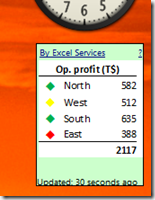For this example, I used a separate table of banding ranges and an Excel VLookup to find the appropriate color for the indictor. Here, 1=green, 2=yellow, 3=red. In the Excel cell I used conditional formatting to choose the text color appropriately. But as we always want to display a certain element (here, the diamond from the WingDings font), I used a custom format for each of the cells, so the number (1, 2 or 3) is not shown but only one character. The corresponding character for the diamond is “u”, so the custom format looks like this: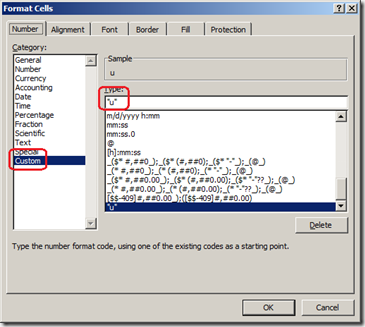And of course, there is a lot more you can do with all these formatting, cube functions etc.

3. Make changes to the source code of the gadget

You can extract the gadget or modify the source code of the gadget yourself. After installing the gadget, the extracted sources can be found here:

After modifying the sources, the gadgets need to be switched off and on in order for the changes to apply. The main file is the gadget.html here. For example you could change the link “By Excel Services” to point on your SharePoint server. To do so, a simple change in the source is needed:

Before:
<tr><td id="dockedTitle" width="100%">
</td><td>

After:
<tr><td id="dockedTitle" width="100%">
http://srv1/PowerPivot/Forms/AllItems.aspx">All reports</a>
</td><td>

I also changed the background color here, so this is how the result looks like:

As you can see, you can do a lot of interesting things with this simple but very powerful desktop gadget.

4. Use parameters

If you want to use the same Excel Services file for more than one user you may want to pass parameters from each individual instance of the desktop gadget to the Excel Services file. This is also possible, however there are some things to take care of.

The syntax for passing a parameter is

Ranges('cellname')=value

Here, cellname is a named cell in Excel that we want to pass the value to. This is how it is entered in the desktop gadget, if our named cell is named ‘value1’ and we want to pass ‘xyz’: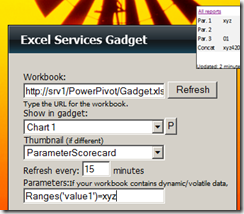Multiple parameters may be passed by separating them with an ampersand, for example

Ranges('value1')=xyz&Ranges('value2')=42&Ranges('value3')=01

This could be our corresponding view in the desktop gadget: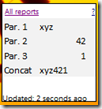I just added a field that concatenates all three parameters to show that the Excel Services sheet is recomputed based on the passed values.

As you can see, all parameters are passed to the gadget. However, numeric parameters are considered as numbers in Excel, so 01 was changed to 1. If you want to prevent this, add a ' in front of each text.

This is how the parameters should look like when you want to make sure, text is passed as text (and not converted to a number or date) in Excel:

Ranges('value1')='xyz&Ranges('value2')=42&Ranges('value3')='01

You should also be aware, that this string is passed to the URL “as it is”. So you have to encode all characters that are not allowed in a URL.

For example, if you want to pass the year 2006 from AdventureWorks, the unique name of the date member would be:

[Date].[Calendar].[Calendar Year].&

In order to pass this to the gadget, you have to encode blanks, the square brackets and the ampersand. So the result would look like this:

Ranges('parDate')=%5BDate%5D.%5BCalendar%5D.%5BCalendar%20Year%5D.%26%5B2006%5D

This is very difficult to read. Therefore I recommend just to pass the key (2006 in this case) as the parameter and to construct the unique name in Excel using a formula like

="[Date].[Calendar].[Calendar Year].&[" & parDate & "]"

Of course, there are a lot more things you can do with this simple, yet powerful Windows desktop gadget. Like with most other gadget you may also place more than one instance of the gadget on your desktop, so you can have different scorecards. You can also add links to your Excel sheet, so that you can jump directly to a dashboard for a specific key performance indicator. Just start playing with the gadget and see how powerful und useful it is.

## Sunday, September 25, 2011

### Custom Aggregates in DAX / BISM Tabular (part 2)

SQL Server Danali | PowerPivot

This post combines two ideas

1. The calculation of a moving average (last 30 days) as shown in a previous post
2. Custom aggregations

What I want to show here today, is how we can influence the way our calculation works on higher levels. For this purpose I’m showing three different approaches:

1. The monthly (quarterly, yearly) total is defined as the last day’s moving average of the given date range.
This corresponds to the original approach from my post about moving averages
2. The monthly (quarterly, yearly) total is defined as the average of all the moving averages on day level.
3. The monthly (quarterly, yearly) total is defined as the average of the sales amount of all days of that period (the moving average is only to be calculated on date level)

Of course, there are many more possibilities but I think the methods shown here, can be used for many other aggregation requirements.

Here are the three aggregations side by side.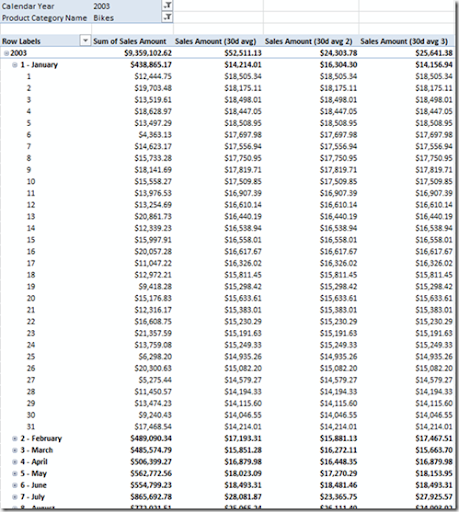Although the values on higher levels of aggregation differ a lot, the daily values are identical (we just wanted to change the rollup method, not the calculation on a detail level).

1. The monthly (quarterly, yearly) total is defined as the last day’s moving average of the given date range

This is shown here for our measure “Sales Amount(30 avg)”: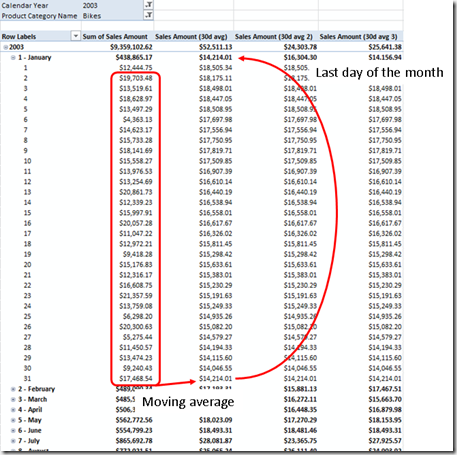As shown in my previous post, the formula may look like this:

Sales Amount (30d avg):=
AverageX(
Summarize(
datesinperiod(
'Date'[Date]
, LastDate('Date'[Date])
, -30
, DAY
)
,'Date'[Date]
, "SalesAmountSum"
, calculate(Sum('Internet Sales'[Sales Amount]), ALLEXCEPT('Date','Date'[Date]))
)
,[SalesAmountSum]
)

Since we use the expression LastDate(‘Date’[Date]) the last date of each period is used for the moving average – exactly what we wanted.

Average at month level as the average of the moving averages (day level)

In this approach, the monthly aggregate has to be calculated as the average of all the daily moving averages of that month. The picture shows what this means: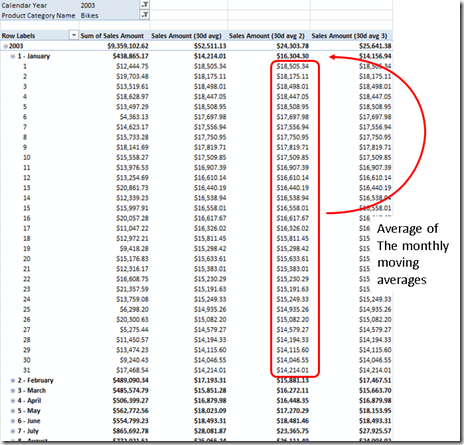This might look pretty difficult. However, for our calculation we simply have to wrap the existing calculation in another Summarize – function.

This is the formula I used:

Sales Amount (30d avg 2):=
AverageX(
summarize(
'Date'
, 'Date'[Date]
, "DayAvg"

AverageX(
Summarize(
datesinperiod(
'Date'[Date]
,LastDate('Date'[Date])
, -30
, DAY
)
,'Date'[Date]
, "SalesAmountSum"
, calculate(Sum('Internet Sales'[Sales Amount]), ALLEXCEPT('Date','Date'[Date]))
)
,[SalesAmountSum]
)
)
,[DayAvg]
)

The blue part of the formula is exactly the same is in the first calculation. We’re just wrapping this in an additional Average function. Why does this still work on a day-level? Simply because the outer average computes the average of a single value in this case.

So, with just a minor change to the formula, we changed the method of aggregation quite a lot.

3. The monthly (quarterly, yearly) total is defined as the average of the sales amount of all days of that period

This sounds quite simple but in this case we have to distinguish two calculation paths:

• day level
• month level and above

The following picture shows the calculation for the monthly aggregate: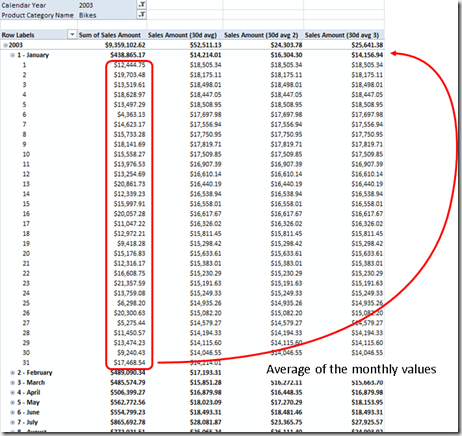In order to split our computation paths we need to find out if we are on day level or not. Usually the IsFiltered(…) DAX function can be used for this purpose. However, since we have some columns with date granularity (date, day of month, day of year) in our date dimension, we would have to write something like IsFiltererd(‘Date’[Date]) || IsFiltered(‘Date’[Day of Month]) || …

To simplify this, I simple used a count of days in the following code. If we count only one day, we’re on day level. Of course, the count is the more expensive operation, but for this example, I leave it that way (the date table is not really big).

Sales Amount (30d avg 3):=
if (
Count('Date'[Date])=1,
AverageX(
Summarize(
datesinperiod(
'Date'[Date]
,LastDate('Date'[Date])
, –30
, DAY
)
, 'Date'[Date]
, "SalesAmountSum"
, calculate(Sum('Internet Sales'[Sales Amount]), ALLEXCEPT('Date','Date'[Date]))
)
,[SalesAmountSum]
)
,
AverageX(
Summarize(
'Date'
,'Date'[Date]
,"SalesAmountAvg"
, calculate(Sum('Internet Sales'[Sales Amount]), ALLEXCEPT('Date','Date'[Date]))
)
,[SalesAmountAvg]
)
)

Again the blue part of the formula is exactly the same as in our first approach. This part is taken whenever we’re on a day level. On higher levels, the aggregate is computed as a simple (not moving) average of all daily values.

So, using the concepts of my previous post we were able to change the aggregation method to meet very sophisticated requirements.

## Sunday, September 11, 2011

### Custom Aggregates in DAX / BISM Tabular (part 1)

SQL Server Denali | PowerPivot

Custom aggregates can be created using cube scripts in BISM multidimensional (SSAS OLAP cubes). How can we do this with BISM tabular? In many cases, simple DAX calculations can solve this for us.

I’m referring to the example of my previous post about semi additive measures. Let’s say we’re monitoring the stock of two products we’re selling. For the totals we want to see the average stock over time. At least once a month we’re taking a snapshot of the stock. If we have more than one snapshot per month, the monthly total computes as the average of those snapshot. For aggregation above the month level we want to take the average of the monthly averages. At first, this looks like we only have to use average as the aggregation function. But the average of averaged values is not identical to the average of all values. Let’s take a look at this source data table: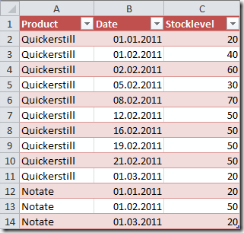For product Notate we have a single measurement of 50 pieces in February. For product Quickerstill we have 8 distinct measurements with an average of 50 pieces in February. However, when we look at the total average for Quickerstill, the 8 distinct measurements in February result in a higher weight of the February average and therefore in a higher total average of 44 instead of 30: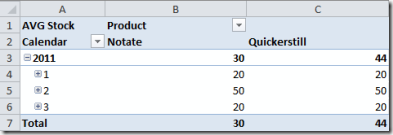The Average Stock measure in this example is the same semi additive measure as in my previous post and computed like this:

AVG Stock:=Sum([Stocklevel])/DISTINCTCOUNT([Date])

The requirement for the custom aggregate means, that we also want to see a total average of 30 for product Quickerstill (20+50+20=90, 90/3=30). This requirement is somewhat unusual as the computation above gives the “correct” average of all values. One interpretation is that the weight for computing the average of two or more months is not influenced by the number of measurements within the month.

We can achieve this in a way that is very similar to the semi additive calculations from my last post. Here is the resulting formula:

Special AVG Stock:=AVERAGEX(SUMMARIZE('Stock',[Year],[Month],"AvgStock",AVERAGE([Stocklevel])),[AvgStock])

This formula simply summarizes the average stock at a grouping level of year and month. Then, in a second step, it takes these values and computes the average of them. By doing so, we have broken the aggregation into two layers. First we average by month, then we take the average of those values.

Here is the resulting table using the new aggregate: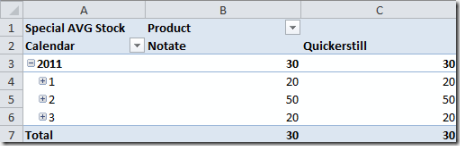And after expanding the February values (2nd month) we clearly see the how our custom aggregate works: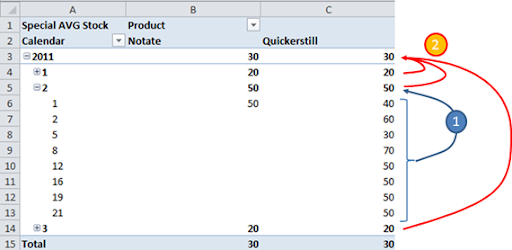Of course, this is just a simple custom aggregate but it is remarkable that we didn’t need any kind of cube script with scope-statements to achieve this but only a very simple DAX expression.

## Sunday, September 4, 2011

### Moving Averages in DAX vs. MDX

SQL Server 2008 | SQL Server 2008R2 | SQL Server Denali | PowerPivot

Yes, I’m a supporter of equal rights of DAX and MDX. And like many others, I can’t wait to have BISM multidimensional (aka OLAP Cubes) supporting DAX so that we can use project Crescent on top of all those nice cubes. But back to my topic. My last post was about moving averages in DAX and I was so sure I blogged about calculating them in MDX before… but I didn’t. This is not fair.

On the other hand, Mosha Pasumansky, the godfather of MDX, wrote an excellent and very complete article about this topic and I can only suggest reading it. It doesn’t only cover simple moving averages but also weighted and exponential ones. Also Bill Pearson wrote a very good step-by-step guide about this topic. You can find it here and I can only suggest reading it.

So, basically there is no need for me to write another article about this. Therefore this will be a very short blog post… ah, I just remembered something I may write about. Mosha and Bill both investigated on the calculation of moving averages within a query. In the context of a specific query, things are sometimes easier compared to the situation where you create a cube measure that has to work under different query conditions. For example, you cannot be sure which hierarchy has been used.

The first thing that comes into mind is the wizard for adding time intelligence. This wizard does a pretty good job. The main result is a short piece of code that is inserted into the cube script. This piece of code looks similar to the following example:

Scope(
{
[Measures].members
}
) ;

/*Three Month Moving Average*/
(
[Date].[Calendar Date Calculations].[Three Month Moving Average],
[Date].[Month Name].[Month Name].Members,
[Date].[Date].Members
)
=
Avg(
ParallelPeriod(
[Date].[Calendar].[Month],
2,
[Date].[Calendar].CurrentMember
)
: [Date].[Calendar].CurrentMember
*
[Date].[Calendar Date Calculations].[Current Date]
) ;
End Scope ;

The result can be intuitively used by end users as they simply have to choose in which kind of view the data should appear (actual, three month moving average or any other calculation generated by the wizard, for example year-to-day or year-over-year growth). Also, this computation is focusing on the data dimension, not the specific measure, so it can be used for any measure in the cube.

In my last post I used a DAX calculation that computed the moving average based on the last date in the current interval. We can do pretty much the same in MDX by “translating” the DAX formula to MDX. Here is the calculation for a cube calculated member:

CREATE MEMBER CURRENTCUBE.[Measures].SalesAmountAvg30d AS
Avg(
LASTPERIODS(
30
, tail(descendants([Date].[Calendar].currentmember,[Date].[Calendar].[Date]),1).item(0)
)
, [Measures].[Internet Sales Amount]
);

After defining this measure we can use it in a query or within a pivot table. Here’s the result from a query:

select {[Measures].[Internet Sales Amount], [Measures].[SalesAmountAvg30d]} on 0,
descendants([Date].[Calendar].[Calendar Year].&)  on 1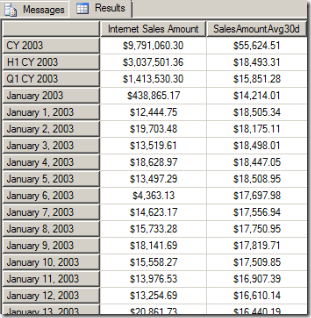If you compare these values with the values from my last post you see that the values are absolutely identical (just the order of the values differs because of the way I wrote the query). Here are both definitions side by side:

 MDX DAX Avg( LASTPERIODS(     30    , tail(        descendants(          [Date].[Calendar].currentmember          ,[Date].[Calendar].[Date]        )        ,1      ).item(0)    )    , [Measures].[Internet Sales Amount] ); AverageX( Summarize(   datesinperiod('Date'[Date]     , LastDate('Date'[Date]),-30,DAY)     ,'Date'[Date]     , "SalesAmountSum"    , calculate(       Sum('Internet Sales'[Sales Amount]),      ALLEXCEPT('Date','Date'[Date])      )    )    ,[SalesAmountSum] )

Again, the idea (approach) is the same in both cases, therefore both definitions are similar. However, in my opinion the DAX syntax is a bit harder to read in this case. Especially the CALCULATE(…, ALLEXCEPT(…)) makes it harder to understand. In MDX, we rely on attribute relationship for this purpose but in DAX we need to “break” the context manually.

Now, let’s do some performance tests. In order to compare performance I used these queries

 MDX DAX with member SalesAmountAvg AS    Avg(     LASTPERIODS(        30       , tail(         descendants(           [Date].[Calendar].currentmember           ,[Date].[Calendar].[Date]),1         ).item(0)     )     , [Measures].[Internet Sales Amount]   )   select {   [Measures].[Internet Sales Amount]   , SalesAmountAvg } on 0, descendants([Date].[Calendar].[All Periods],,LEAVES) on 1 from [Adventure Works] define measure 'Internet Sales'[SalesAmountAvg] =   AverageX(     Summarize(       datesinperiod('Date'[Date]        , LastDate('Date'[Date]),-30,DAY)        ,'Date'[Date]        , "SalesAmountSum"       , calculate(            Sum(           'Internet Sales'[Sales Amount])           ,ALLEXCEPT(           'Date','Date'[Date])         )     )    ,[SalesAmountSum] ) evaluate (   addcolumns(     values('Date'[Date])     ,"Internet Sales Amount"     , SumX(relatedtable        ('Internet Sales'),[Sales Amount])     ,"SalesAmountAvg",     'Internet Sales'[SalesAmountAvg]   ) )

Both queries return exactly the same results (you may add an “order by ‘Date’[Date]” at the end of the DAX query in order to have the dates returned in the same order as from the MDX query).

For the MDX queries I cleared the cache before running the queries. I changed the number of days (number of days to include in the average, written bold, red in the queries above) and got the following results. For number of days = 0 I took out the calculation and left only the sales amount as aggregate. Time was measured in seconds using SQL Server Management Studio (on a virtual machine, old hardware).

 n=0 n=10 n=30 n=50 n=100 n=1000 MDX 1 3 3 3 3 4 DAX 0 9 9 9 9 12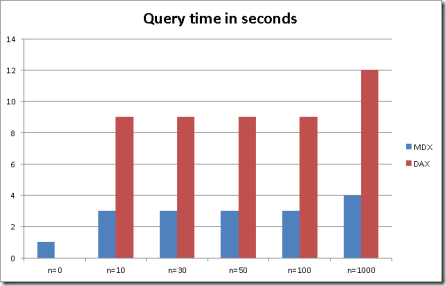When looking at these results I was somewhat surprised. Not about the situation that the DAX query took longer to execute. Please keep in mind that I’m running the queries on an early preview of the product so I suppose there is still a lot of debugging and internal logging going on here. We will have to wait for the final product to make a comparison. What surprises me was the fact that the DAX query time did not go up significantly with higher values of n. For the MDX engine I was pretty sure that it would perform this way because we have mature and a well built cache behind it. So, although we’re increasing the number of computed cells dramatically (with higher values for n), the MDX query performance should almost be constant as we have a lot of overlapping calculations here. But also the current DAX engine performs in the same way that shows how very well the DAX engine is implemented. This is a pretty good result and we can expect a lot of performance from this new DAX query engine.

## Sunday, August 21, 2011

### Moving Average in DAX / BISM Tabular

SQL Server Denali | PowerPivot

Alberto Ferrari already wrote about calculating moving averages in DAX by using a calculated column. I’d like to present a different approach here by using a calculated measure. For the moving average I’m calculating a daily moving average (over the last 30 days) here.

For my example, I’m using the PowerPivot workbook which can be downloaded as part of the SSAS Tabular Model Projects from the Denali CTP 3 samples.

In this post, I’m developing the formula step by step. However, if you are in a hurry, you might directly want to jump to the final results below.

With calendar year 2003 on the filter, date on columns and sales amount (from table Internet Sales) in the details, the sample data looks like this: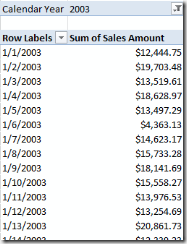In each row’s context, the expression 'Date'[Date] gives the current context, i.e. the date for this row. But from a calculated measure we cannot refer to this expression (as there is no current row for the Date table), instead we have to use an expression like LastDate('Date'[Date]).

So, in order to get the last thirty days we can use this expression

DatesInPeriod('Date'[Date],LastDate('Date'[Date]),-30,DAY)

We can now summarize our internet sales for each of those days by using the summarize function:

Summarize(
DatesInPeriod('Date'[Date],LastDate('Date'[Date]),-30,DAY)
,'Date'[Date]
, "SalesAmountSum"
, Sum('Internet Sales'[Sales Amount])
)

And finally, we’re using the DAX function AverageX to compute the average of those 30 values:

Sales Amount (30d avg):=AverageX(
Summarize(
DatesInPeriod('Date'[Date],LastDate('Date'[Date]),-30,DAY)
,'Date'[Date]
, "SalesAmountSum"
, Sum('Internet Sales'[Sales Amount])
)
,[SalesAmountSum]
)

This is the calculation that we are using in our Internet Sales table as shown in the screenshot below: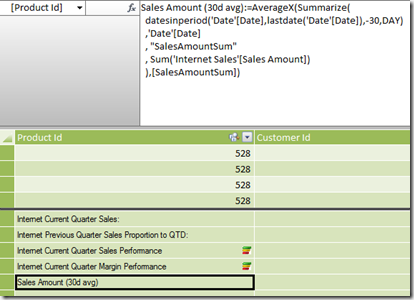When adding this calculation to the pivot table from above, the result looks like this: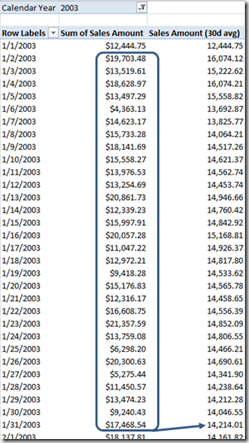Looking at the result it seems that we don’t have any data prior to January 1, 2003: The first value for the moving average is identical to the day value (there are no rows before that date). The second value for the moving average is actually the average of the first two days and so on. This is not quite correct but I’m getting back to this problem in a second. The screenshot shows the computation for the moving average of at January 31 as the average of the daily values from January 2 to 31.

Our calculated measure also works fine when filters are applied. In the following screenshot I used two product categories for the data series: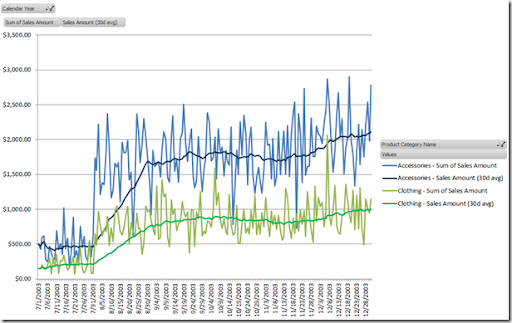How does our calculated measure work on higher aggregation levels? In order to find out, I’m using the Calendar hierarchy on the rows (instead of the date). For simplicity I removed the semester and quarter levels using Excel’s pivot table options (Show/Hide fields option).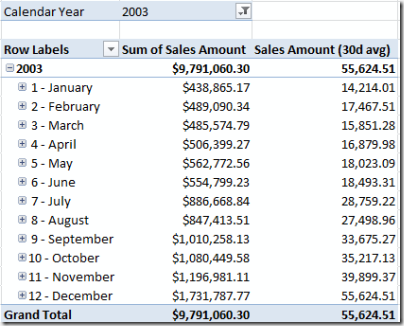As you can see, the calculation still works fine. Here, the monthly aggregate is the moving average for the last day of the specific month. You can see this clearly for January (value of 14,215.01 also appears in the screenshot above as the value for January 31). If this was the business requirement (which sounds reasonable for a daily average), then the aggregation works fine on a monthly level (otherwise we will have to fine tune our calculation and this will be a topic of am upcoming post).

But although the aggregation makes sense on a monthly level, if we expand this view to the day level you’ll see that our calculated measure simply returns the sales amount for that day, not the average of the last 30 days anymore: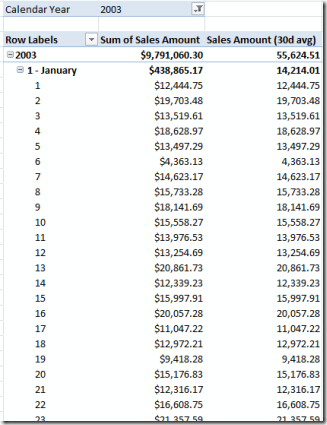How can this be. The problem results from the context in which we calculate our sum, as highlighted in the following code:

Sales Amount (30d avg):=AverageX(
Summarize(
datesinperiod('Date'[Date],LastDate('Date'[Date]),-30,DAY)
,'Date'[Date]
, "SalesAmountSum"
, Sum('Internet Sales'[Sales Amount])
)

,[SalesAmountSum]
)

Since we evaluate this expression over the given dates period, the only context that is overwritten here, is 'Date'[Date]. In our hierarchy we’re using different attributes from our dimension (Calendar Year, Month and Day Of Month). As this context is still present, the calculation is also filtered by those attributes. And this explains why we the current day’s context is still present for each line. To get things clear, as long as we evaluate this expression outside of a date context, everything is fine as the following DAX query shows when being executed by Management Studio on the Internet Sales perspective of our model (using the tabular database with the same data):

evaluate (
Summarize(
datesinperiod('Date'[Date],date(2003,1,1),-5,DAY)
,'Date'[Date]
, "SalesAmountSum"
, Sum('Internet Sales'[Sales Amount])
)
)

Here, I reduced the time period to 5 days and also set a fixed date as LastDate(…) would result in the last date of my date dimension table for which no data is present in the sample data. Here is the result from the query: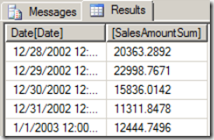However, after setting a filter to 2003, no data rows outside of 2003 will be included in the sum. This explains the remark above: It looked like we only have data starting from January 1, 2003. And now, we know why: The year 2003 was on the filter (as you can see in the very first screen shot of this post) and therefore it was present when calculating the sum. Now, all we have to do is to get rid of those additional filters because we’re already filtering our results by Date. The easiest way to do so, is to use the Calculate function and apply ALL(…) for all attributes for which we want to remove the filter. As we have some of those attributes (Year, Month, Day, Weekday, …) and we want to remove the filter from all of them but the date attribute, the shortcut function ALLEXCEPT is very useful here.

If you do have an MDX background you will wonder why we don’t get a similar problem when using SSAS in OLAP mode (BISM Multidimensional). The reason is that our OLAP database has attribute relationships, so after setting the date (key) attribute, the other attributes are automatically changed too and we don’t have to take care about this (see my post here). But in the tabular model we don’t have attribute relationships (not even a true key attribute) and therefore we need to eliminate unwanted filters from our calculations.

So here we are with the …

Final results

Sales Amount (30d avg):=AverageX(
Summarize(
datesinperiod('Date'[Date],LastDate('Date'[Date]),-30,DAY)
,'Date'[Date]
, "SalesAmountSum"
, calculate(Sum('Internet Sales'[Sales Amount]), ALLEXCEPT('Date','Date'[Date]))
)
,[SalesAmountSum]
)

And this is our final pivot table in Excel: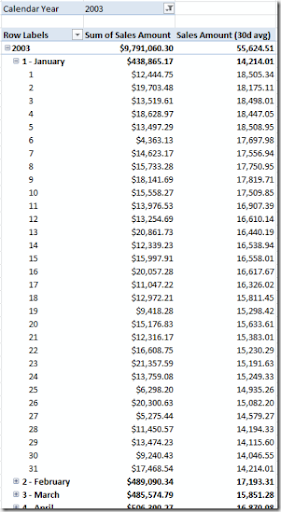To illustrate the moving average, here is the same extract of data in a chart view (Excel):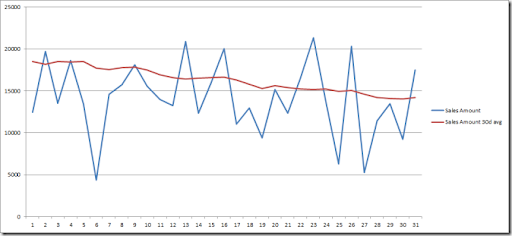Although we filtered our data on 2003 the moving average for the first 29 days of 2003 correctly takes the corresponding days of 2002 into account. You will recognize the values for January 30 and 31 from our first approach as these were the first days for which our first calculation had a sufficient amount of data (full 30 days).

## Saturday, August 13, 2011

### Semi additive measures in DAX / BISM Tabular

SQL Server Denali | PowerPivot

Semi additive measures, i.e. measures that have to be aggregated differently over different dimensions, are commonly used in BI solutions. One example could be stock levels. Of course we don’t want to sum them up over time, but only over product, location etc. For the time, a different aggregation is used, for example average or last value.

The following example shows how to implement some of the most commonly used semi additive measures in DAX.

In my example I’m using PowerPivot (Denali edition), but the same calculations can be used in a BISM Tabular model in Visual Studio.

In order to keep things simple, I’m using just a short table of test data: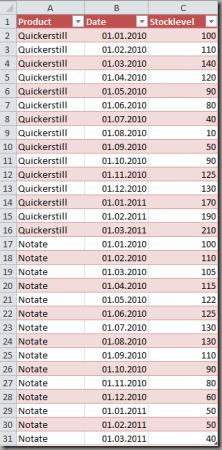As you see, we only have two products with monthly stock levels in 2010 and 2011.

Although not needed for my semi additive measures, I created additional columns in my PowerPivot sheet for convenient reasons: Year, Month, Day (using the corresponding DAX-function with the same name). I also set the newly created columns, as well as the Stocklevel column to hidden (it makes no sense to sum up the stock level). Although the date information is kept in the same table as the data to keep things simple for this example, I encourage to build a separate date dimension table here (similar idea as with a date dimension in a multidimensional model).

Finally, I created a hierarchy named ‘Calendar’ on my newly created date columns: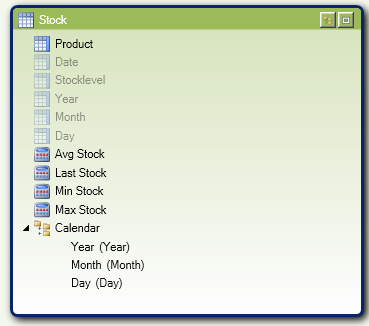Average (over time)

Let’s start with an easy one, the average over time. Since we can easily compute the distinct count of our date values, we can simply add up the stock level and divide it by the distinct count. In my example the formula looks like this:

Avg Stock:=Sum([Stocklevel])/DISTINCTCOUNT([Date])

Last value (over time)

In order to compute the last value, the DAX function LASTDATE comes in handy. Here is the formula:

Last Stock:=CALCULATE(SUM([Stocklevel]),LASTDATE('Stock'[Date]))

Min/Max value (over time)

For min/max we have to be a little bit more tricky. In the approach I’m showing here, I’m grouping the table by date by using the SUMMARIZE function and the SUM aggregation. Then I’m using the function MINX or MAXX to find the minimal or maximal value.

Here are the two formulas:

Max Stock:=MINX(SUMMARIZE('Stock','Stock'[Date],"SumByDate",SUM('Stock'[Stocklevel])),[SumByDate])

Max Stock:=MAXX(SUMMARIZE('Stock','Stock'[Date],"SumByDate",SUM('Stock'[Stocklevel])),[SumByDate])

To understand these formulas you can see the effect pretty well after restoring the PowerPivot workbook to a SSAS server in tabular mode. After doing so, we can create a query to show the result of the inner SUMMARIZE function using this DAX query:

evaluate(
SUMMARIZE('Stock','Stock'[Date],"SumByDate",SUM('Stock'[Stocklevel]))
)

Here’s the result: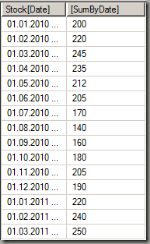The MinX or MaxX function simply takes the lowest/highest value from this table.

Now let’s see, how this looks like in Excel. The following screenshot shows the calculations in my PowerPivot sheet: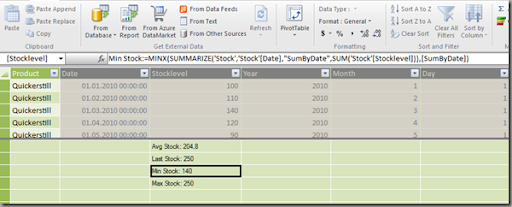Here’s the result in Excel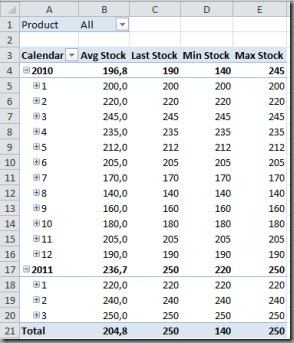And of course, the aggregations also work correctly when filtering the data as shown below (single select on product and multi select on months):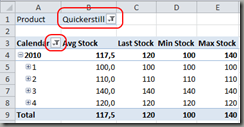Another cool feature is that besides DAX we can still use standard MDX to query our SSAS tabular model, for example:

select
{[Measures].[Avg Stock],[Measures].[Last Stock],
[Measures].[Min Stock],[Measures].[Max Stock]} on 0,
[Stock].[Calendar].[Year] on 1
from [Model]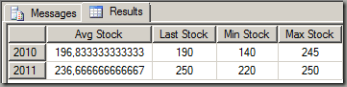A final word about empty (missing) rows: The above calculations need a value of zero as the information that there is no stock at that month. If the value is left blank (no source data row at all), the month itself is treated as missing (interpretation more like we didn’t have this product in our portfolio at all).

## Saturday, July 30, 2011

### Parallel hierarchies in a parent-child structure

SQL Server 2005 | SQL Server 2008 | SQL Server 2008R2

This post is about a problem I faced some years ago. The source system was SAP with user defined hierarchies, in this case within the cost center and cost type tables. Parallel hierarchies are well supported in SQL Server BI but in this case, users in SAP could define multiple hierarchies on their own and they wanted these hierarchies to be also available in the OLAP cube. For example, costs associated with the cost center 1000 should be analyzed as shown below: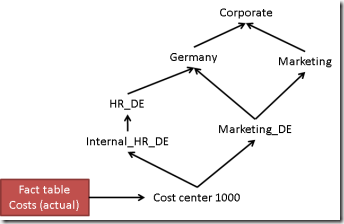All costs that are booked on cost center 1000 have to appear in the hierarchy as shown in the sketch. And end-users may also be able to create new hierarchies (for example to analyze a certain project). Of course there may be better ways to model this but in this case we had basically two tables for the cost centers:

• table CC (Cost Center)
• table CCG (Cost Center Group)

Table CC contains all cost centers (for example the above cost center 1000) together with additional information (like name, responsible person etc.) while table CCG contains the hierarchy. in CCG we basically find two columns:

• Node name
• Parent node name
 Node Parent Node 1000 Internal_HR_DE Internal_HR_DE HR_DE HR_DE Germany 1000 Marketing_DE Marketing_DE Germany Germany Corporate Marketing_DE Marketing Marketing Corporate

Facts (in this case actual or planned costs) are associated with the cost center number (for example 1000). Usually, parent-child hierarchies may be used in this case where we have a very dynamic structure and we do not know the number of levels. However, parent-child may only be used if each node has at most one parent. But here we find the cost center 1000 having two parents (Internal HR Costs DE and Marketing_DE). The same situation exists with the Marketing_DE node (having parents Marketing and Germany).

The solution I’m presenting here is to create additional nodes until each node only has one parent. This is possible as each node of a parent-child hierarchy in SSAS has a name and a key property. So, the name will be identical, while the key will be different. In order to show the process, let’s add internal keys to each of the hierarchy elements.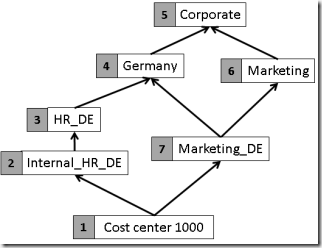What we have to do now is to create additional nodes for every node that has more than one parent. Let’s start with the ‘Marketing_DE’ node: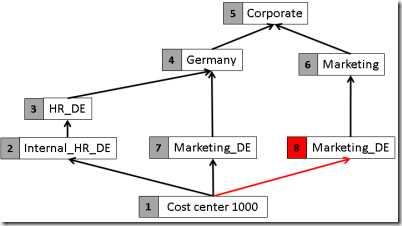The additional node gets a new (internal) key, in this example the number 8. But there is still a node with multiple parents: the cost center 1000. Let’s also transform this into separate nodes: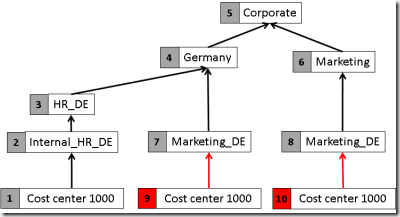After this step, each node has at most one parent and therefore the structure can be modeled as an SSAS parent-child hierarchy.

But now, we have to think about the fact rows. Without the hierarchy, facts would have been associated to the cost center by using the internal key (surrogate key), so for example 1000 € that are booked on cost center 1000 would appear in the fact table like

 DateKey CostCenterKey … Amount … … … … 20110630 1 … 1000 … … … …

But now, we have to associate this single fact row to three rows in the dimension table (as the cost center 1000 appears three times now). Therefore we have to use a many-to-many approach, so we add another table, a so called bridge table with the following rows:

 CostCenterKey CostCenterDimKey … … 1 1 1 9 1 10 … …

For technical reasons, our fact table has to be linked to a dimension (of flat cost centers), which is also used by the bridge table. This is shown in the following image: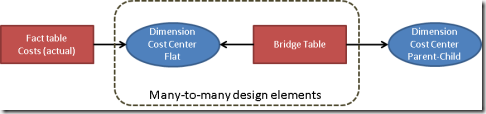The most difficult part here is to “normalize” the parent-child structure. One way to do this is to use a stored procedure. Here is the code I used. Within this procedure, the following tables are used:

masterdata.Costcenter the flat table of cost centers (only leaf-level). The key field is the cost center number (for example 1000 for our cost center from above)
masterdata.CostcenterGroup the hierarchy structure as shown above
ods.CostcenterGroupExpanded Output table: the expanded tree containing the fields of the table masterdata.Costcenter plus the following additional fields:

 CostcenterKey the new generated surrogate key ParentKey the key of the parent node Level a technical field used during iteration

Here is the code:

CREATE PROCEDURE [dbo].[ExpandCostcenterGroup]
AS
SET NOCOUNT ON
truncate table ods.costcenterGroupExpanded

declare @level int
declare @affectedrows int
declare @totalrowcount int

set @level=0

insert into ods.costcenterGroupExpanded(costcentergroup,Parentgroup,Description1,Description2,Responsibility,AccountingArea)
Select distinct costcenterGroup, Parent,Description1,Description2,Responsibility,AccountingArea from masterdata.costcentergroup

-- Initialize all keys
update ods.costcenterGroupExpanded
set ParentKey=(select min(costcenterkey) from ods.costcenterGroupExpanded where costcenterGroup=c.Parentgroup)
from ods.costcenterGroupExpanded as c
where not c.ParentGroup is null

set @affectedrows=1

while @affectedrows>0
begin

Set @level=@level+1

set @totalrowcount=(select Count(*) from ods.costcenterGroupExpanded)

insert into  ods.costcenterGroupExpanded(costcentergroup,Parentgroup,ParentKey,"level",Description1,Description2,Responsibility,AccountingArea)
select distinct cparent.costcentergroup, cparent.Parentgroup,cparent.costcenterKey,@level,cparent.Description1,cparent.Description2,cparent.Responsibility,cparent.AccountingArea
from ods.costcenterGroupExpanded as cparent inner join ods.costcenterGroupExpanded as cchild on
cparent.Parentgroup=cchild.costcenterGroup
where cparent.ParentKey!=cchild.costcenterKey
and cchild."Level"=@level-1

set @affectedrows=@@rowcount

end

return

GO

To keep things simple, I truncate the output table CostcenterGroupExpanded here. However, there is a drawback with this approach: The surrogate keys may change after changes of the imported source tables. This will result in a problem for example for the Excel users. If you’re using filters like ‘show this element only’, only the key is stored.

In order to avoid this you will need to store the mapping and the assigned surrogate key separately. Here it is necessary not only to store the combination of cost center/parent cost center/surrogate key but the whole branch up to the root instead. If you look at the example above you will find two entries of ‘Cost Center 1000’ –> ‘Marketing_DE’, so this is not unique. You have to store the full path up to the root for each node (not only for the leaf-nodes) to make it unique:

 Path Given Surrogate Key 1000 –> Internal_HR_DE –> HR_DE –> Germany –> Corporate 1 1000 –> Marketing_DE –> Germany –> Corporate 9 1000 –> Marketing_DE –> Marketing –> Corporate 10 Marketing_DE –> Germany –> Corporate 7 Marketing_DE –> Marketing –> Corporate 8 …

In order to store the full path up to the root level I recommend using a hash code (MD5 for example) as this is easier to handle as a long list of node names. In this case our additional key store table would look like this

 PathMD5 Given Surrogate Key 417913d10ef49f5ff90db9db9f3d2569 1 8e27be6b156a52016e01dc049bc39126 9 52b1bcaec016e09d4086f37e63814aa5 10 …

The sample code above does not manage this key store table so the keys may change a lot on each load. But for practical purposes you will have to add this key management to make sure the same node always gets the same key.